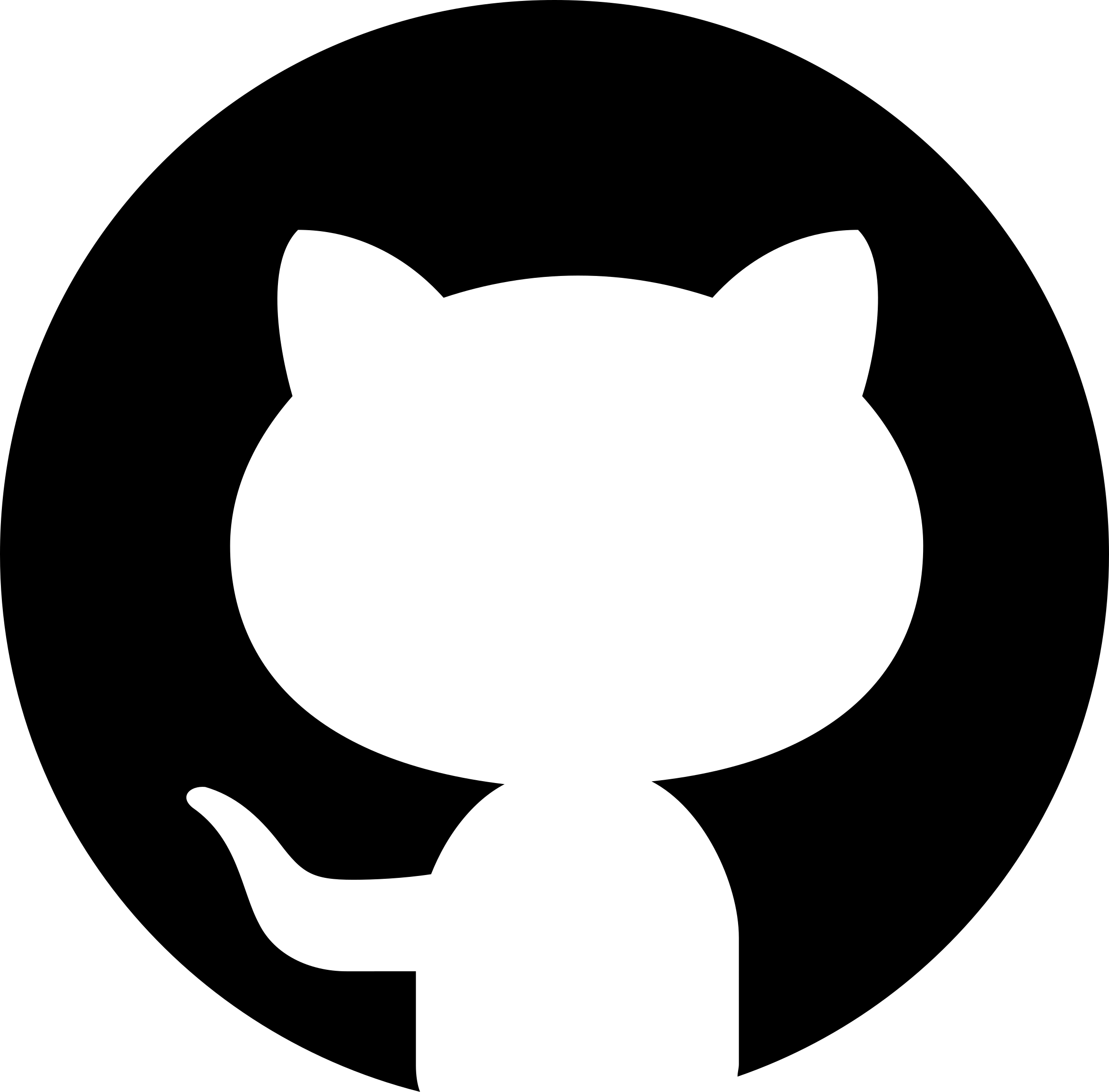# Statistical Process MonitoringView on GitHub

# Statistical Process Monitoring

The purpose of Statistical Process Monitoring is to determine if the performance of a process is maintaining an acceptable level of quality.

Any process will experience natural variability, that is, variability due to essentially unimportant and uncontrollable sources of variation.

A process may experience more serious types of variability in key performance measures (Significant variability).

Sources of variability may arise from one of several types of non-random “causes,” such as operator errors or improperly adjusted dials on a machine.

## Variability

A production process is often subject to variability. There are 2 types:

• variability due the effect of many small, essentially unavoidable causes (a process that only operates with such common causes is said to be in (statistical) control)
• variability due to special causes, such as improperly adjusted machines, operator errors, defective materials, etc. (the variability is typically much larger than for common causes, and the process is said to be out of (statistical) control)

The aim of statistical process monitoring (SPM) is to identify occurrence of special causes.

# Time Series

So far, we have treated samples ${X_1, ... , X_n}$ as if they arose as a result of a random experiment, i.e. $X_i$ is drawn from some distribution with population mean $\mu$ and population variance $σ^2$, and we use $\overline{X}$ and $S^2$ as estimates of $\mu$ and $σ^2$.

In practice, the index $i$ is often a time index, which is to say that the $X_i$ are observed in sequence. In this case, we say that the sample is a time series.

If distribution changes over time due to external factors (war, pandemic, election, etc.) or internal factors (modification of the manufacturing process, policy change, etc.), the sample mean and the sample variance might not provide a useful summary of the situation.

To get a sense of what is going on, it is preferable to plot the data in the order that it has been collected, where the horizontal coordinate is the time of collection $t$ (order, day, week, quarter, year, etc.) and the vertical coordinate is the observation $x_t$. We look for trends, cycles, shifts, etc.

# Control Charts

A control chart consists of observed values of a statistic, such as $\overline{x}$ or $s$, plotted as a time series.

If the true mean $\mu$ and the true standard deviation $\sigma$ of the process are known, then the CLT implies that

$\overline{X}_i \sim N(\mu, \sigma^2/n)$ for all $i$

and one would expect that the observed sample means $\overline{x}$ would lie in the interval

$\mathrm{LCL} = \mu - z_{\alpha/2}\frac{\sigma}{\sqrt{n}}$
$\mathrm{UCL} = \mu + z_{\alpha/2}\frac{\sigma}{\sqrt{n}}$

roughly $100(1-\alpha)%$ of the time.

The upper control limit (UCL) is the upper end of the interval, the lower control limit (LCL) is the lower end of the interval, and the central line (CL) is $\mu$.

For such charts, if we observe $x_i > \mathrm{UCL}$ or $x_i < \mathrm{LCL}$, we have an indication that the process is instable and potentially out of (statistical) control.

The parameter $α$ is again interpreted as the probability of a type I error:

$α = P$(signal of instability | process is stable).

Typically, we use $z_{α/2} = 3$, i.e. $α = 0.9973$. If $N ≤ 30$, that means that even one value outside the control limits is enough to make us suspect that something is off.

In practice, however, $\mu$ and $σ^2$ are not known. In that case, we estimate $\mu$ by the observed grand mean $x$, $3\frac{\sigma}{\sqrt{n}}$ with the help of the observed mean of standard deviations $\overline{s}$:

$\mu \approx \overline{x}, \ 3\frac{\sigma}{\sqrt{n}} \approx A_3(n)\overline{s}$

In this case, the UCL, LCL, and CL are, respectively:

$\mathrm{UCL} = \overline{x} + A_3(n)\overline{s}, \ \mathrm{LCL} = \overline{x} - A_3(n)\overline{s}, \ \mathrm{CL} = \overline{x}$

## Reprise

A control chart consists of:

• points representing a sample statistic taken from the process at different times
• the grand mean and the mean standard deviation of the sample statistic, which is computed using all observations, and is used to determine
• the center line, which is draw at the value of the grand mean
• the upper and lower control limits which indicate the threshold at the which the process output is considered statistically unlikely (typically three standard deviations away from the central line).